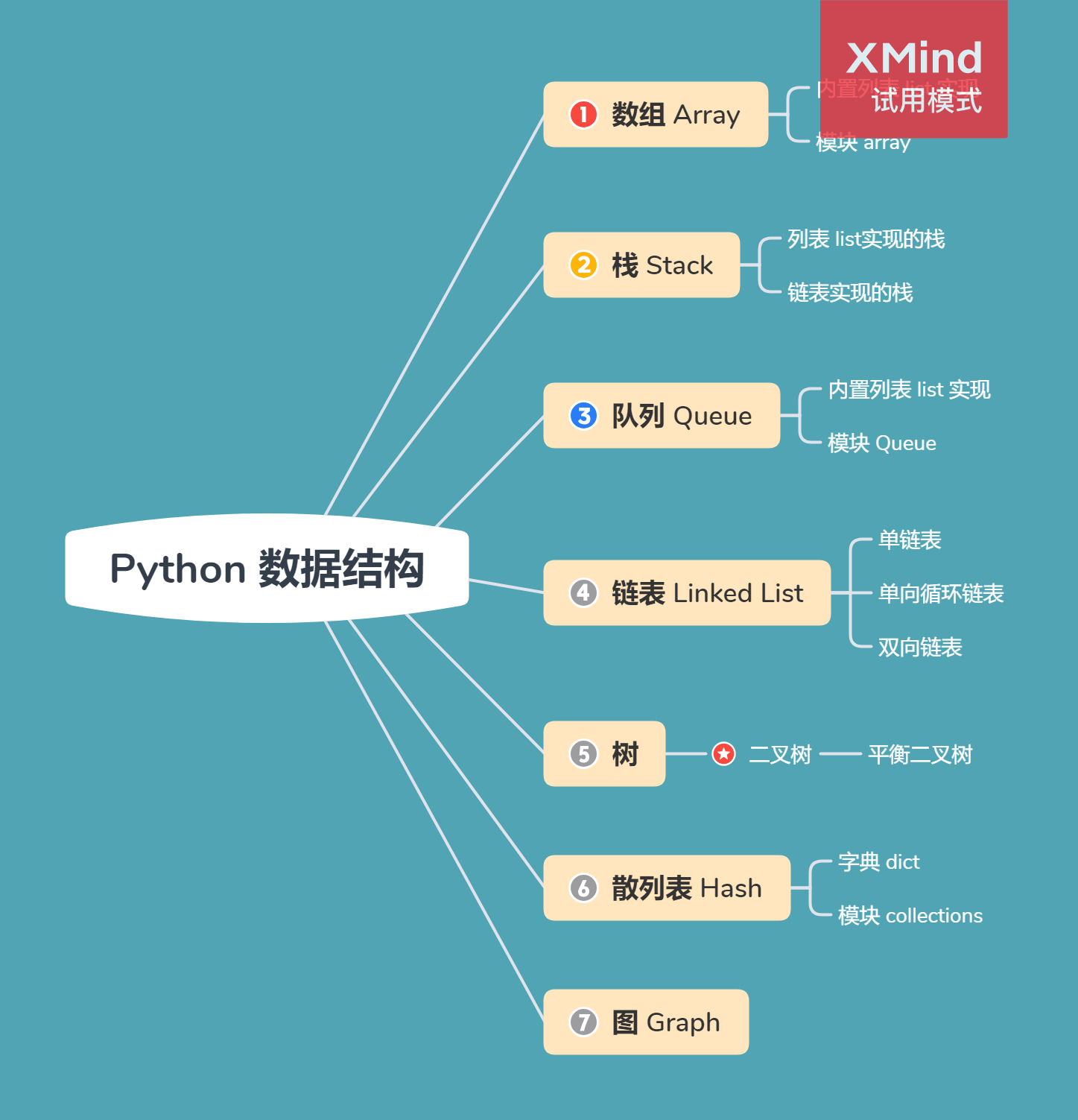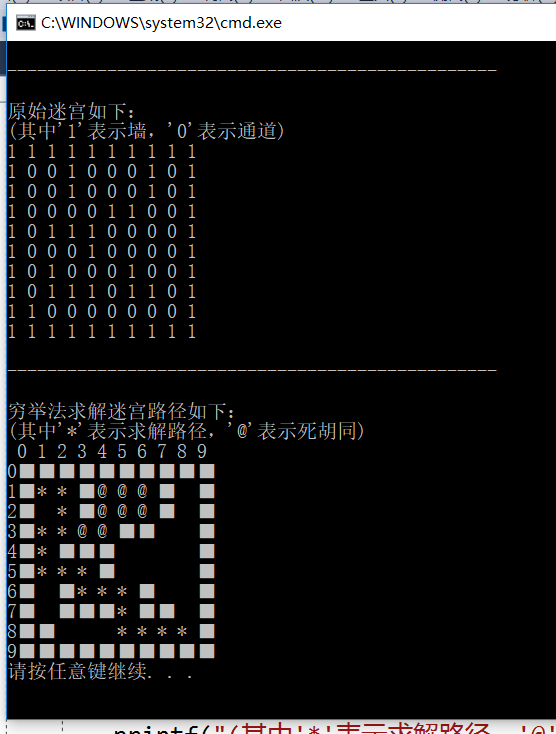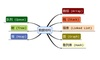• 数据结构迷宫求解代码
千次阅读
2018-06-15 17:01:40
#include "iostream"
#include "malloc.h"
#define TRUE 1;
#define FALSE 0;
#define INIT_STACK_SIZE 20
#define INCREATE_STACK_SIZE 10
typedef int Status;
using namespace std;

//位子坐标
struct Position
{
int x;
int y;
};

typedef struct
{
Position pos;
int di;
}SElemType;

typedef struct
{
SElemType * base;
SElemType * top;
int sizeStack;
}MazeStack;

Position BeginPos;
Position EndPos;
int w, h;;

Status InitStack(MazeStack & maze)
{
maze.base = (SElemType *)malloc(sizeof(SElemType) * INIT_STACK_SIZE);
if (maze.base == NULL)
return FALSE;
maze.top = maze.base;
maze.sizeStack = INIT_STACK_SIZE;
return TRUE;
}

Status IsEmpty(MazeStack & maze)
{
if (maze.base == maze.top)
return TRUE;
return FALSE;
}

Status Push(MazeStack & maze, SElemType & ele)
{
if (maze.base - maze.top >= maze.sizeStack)
{
maze.base = (SElemType *)realloc(maze.base, sizeof(SElemType) * (INCREATE_STACK_SIZE + maze.sizeStack));
if (maze.base == NULL)
return FALSE;
maze.top = maze.base + maze.sizeStack;
maze.sizeStack = maze.sizeStack = INCREATE_STACK_SIZE;
}

*maze.top = ele;
maze.top++;
return TRUE;
}

Status Pop(MazeStack & maze, SElemType & ele)
{
if (maze.base == maze.top)
return FALSE;
ele = *(--maze.top);
return TRUE;
}

Status GetTail(MazeStack & maze, SElemType & ele)
{
if (IsEmpty(maze))
return FALSE;
ele = *(maze.top - 1);
return TRUE;
}

//判断是否是墙
Status Pass(int **a, Position & pos)
{
if (a[pos.x][pos.y] == 0)
return FALSE;
return TRUE;
}

//走过的话，标记为2
void FootPrint(int ** a, Position & pos)
{
a[pos.x][pos.y] = 2;
}

//设置为墙
void MarkPrint(int ** a, Position & pos)
{
a[pos.x][pos.y] = 0;
}

//判断是否走过
SElemType  NextPos(int **a, SElemType & ele)
{
switch (ele.di)
{
//向东寻找
case 1:
ele.pos.x++;
if (a[ele.pos.x][ele.pos.y] != 2)
break;
ele.pos.x--;//继续寻找下去
//向南寻找
case 2:
ele.pos.y++;
if (a[ele.pos.x][ele.pos.y] != 2)
break;//继续寻找下去
ele.pos.x--;
//向西寻找
case 3:
ele.pos.x--;
if (a[ele.pos.x][ele.pos.y] != 2)
break;
ele.pos.x++;
//最后一个方向，如果走过的话，那么必然是死路，那么就将其变成墙
case 4:
ele.pos.y--;
if (a[ele.pos.x][ele.pos.y] == 2)
{
MarkPrint(a, ele.pos);//设为墙
ele.pos.y++;
}
break;
}
return ele;
}

Status CreateMaze(int ** & a)//如果不传引用的话，就应该传*** a
{

int temp;
cout << "请输入迷宫的长，宽: ";
cin >> w;
cin >> h;

cout << "请输入迷宫: " << endl;

a = (int **)malloc(sizeof(int *) * h);
if (a == NULL)
{
cout << "创建迷宫失败!" << endl;
return FALSE;
}
for (int i = 0; i < h; i++)
{
a[i] = (int *)malloc(sizeof(int) * w);
if (a[i] == NULL)
{
cout << "创建迷宫失败!" << endl;
return FALSE;
}
for (int j = 0; j < w; j++)
{
scanf_s("%d", &temp);
a[i][j] = temp;
}
}

cout << "请输入入口:" << endl;
cin >> BeginPos.x;
cin >> BeginPos.y;

cout << "请输入出口:" << endl;
cin >> EndPos.x;
cin >> EndPos.y;

cout << "迷宫创建成功!" << endl;
for (int i = 0; i < h; i++)
{
for (int j = 0; j < w; j++)
cout << a[i][j] << " ";
cout << endl;
}
}

void DistroyMaze(int ** &a)
{
for (int i = 0; i < h; i++)
delete a[i];
delete a;
}

void MazePath(int ** a, MazeStack & mazeStack)
{
SElemType ele;
ele.pos.x = BeginPos.x;
ele.pos.y = BeginPos.y;
ele.di = 1;

do
{
if (Pass(a, ele.pos)) //如果不是墙
{
ele.di = 1;//如果不是墙的话， 就默认寻找东方
Push(mazeStack, ele); //压栈
FootPrint(a, ele.pos);//标记走过
if (ele.pos.x == EndPos.x && ele.pos.y == EndPos.y) //如果是出口，不再循环
break;
ele = NextPos(a, ele); //寻找东方是否走过
}

else //如果是墙
{
if (!IsEmpty(mazeStack))
{
Pop(mazeStack, ele);//返回上一步
while (ele.di == 4 && !IsEmpty(mazeStack)) //如果回路上有好几个格子的四周都是探索过的那么，都在迷宫中这些格子置为墙壁
{
MarkPrint(a, mazeStack.top->pos);
Pop(mazeStack, ele);
}
if (ele.di < 4)
{
ele.di++;
Push(mazeStack, ele); //如果四个方向没有检查完，把刚出栈的重新入栈
ele = NextPos(a, ele);
}
}
}

if (ele.di == 4 && IsEmpty(mazeStack))
{
cout << "该迷宫没有出路!" << endl;
break;
}
} while (true);
}

//输出倒着了
void ShowMaze(int ** & a, MazeStack & mazeStack)
{
SElemType ele;
while(!IsEmpty(mazeStack))
{
Pop(mazeStack, ele);
cout << "( " << ele.pos.x << "," << ele.pos.y << " )  ";
}
}

int main()
{
MazeStack mazeStack;
InitStack(mazeStack);
int ** a = NULL;
CreateMaze(a);
cout << "---------------------------------" << endl;
MazePath(a, mazeStack);
ShowMaze(a, mazeStack);
DistroyMaze(a);
system("pause");
return 0;
}
/*
测试数据：
1 1
3 3
0 0 0 0 0
0 1 1 1 0
0 1 0 0 0
0 1 1 1 0
0 0 0 0 0

*/

更多相关内容
• C语言 数据结构求解迷宫问题实现方法  在学习数据结构栈的这一节遇到了求迷宫这个问题，拿来分享一下~  首先求迷宫问题通常用的是“穷举求解” 即从入口出发，顺某一方向试探，若能走通，则继续往前走，否则...
• 常见的例题有下面四种，会依次进行讲解，本章只讲迷宫求解。 括号匹配：请看数据结构 03-栈的应用：括号匹配的代码实现_江南野栀子的博客-CSDN博客 数制转换：请看数据结构 03-栈的应用：数制转换的代码实现_江...目       录

1. 栈的应用场景

1.1 实际应用场景

1.2 例题分类

2. 应用场景：迷宫求解

2.1 迷宫求解例题

2.2 迷宫求解代码实现

# 1. 栈的应用场景

## 1.1 实际应用场景

总的来说，栈的运用还是非常广泛的，在实际的编程场景中，支持文本编辑器、字处理程序、电子表格程序、绘图程序或类似的应用程序中的撤销功能，支持维护 Web 浏览器所访问过的连接的历史记录。

## 1.2 例题分类

常见的例题有下面四种，会依次进行讲解，本章只讲迷宫求解。

# 2. 应用场景：迷宫求解

## 2.1 迷宫求解例题

例题内容：

给你一个 m x n 的迷宫矩阵 maze （下标从 0 开始），矩阵中有空格子（用 '.' 表示）和墙（用 '+' 表示）。同时给你迷宫的入口 entrance ，用 entrance = [entrancerow, entrancecol] 表示你一开始所在格子的行和列。

每一步操作，你可以往 上，下，左 或者 右 移动一个格子。你不能进入墙所在的格子，你也不能离开迷宫。你的目标是找到离 entrance 最近 的出口。出口 的含义是 maze 边界 上的 空格子。entrance 格子 不算 出口。

请你返回从 entrance 到最近出口的最短路径的 步数 ，如果不存在这样的路径，请你返回 -1 。

## 2.2 迷宫求解代码实现

下面是比较官方的一个代码实现，使用了 collections 中的 deque 结构。

def nearestExit(maze, entrance) :
m, n = len(maze), len(maze)
# 上下左右四个相邻坐标对应的行列变化量
dx = [1, 0, -1, 0]
dy = [0, 1, 0, -1]
# 入口加入队列并修改为墙
q = deque([(entrance, entrance, 0)])
maze[entrance][entrance] = '+'
while q:
cx, cy, d = q.popleft()
# 遍历四个方向相邻坐标
for k in range(4):
nx = cx + dx[k]
ny = cy + dy[k]
if 0 <= nx < m and 0 <= ny < n and maze[nx][ny] == '.':
# 新坐标合法且不为墙
if nx == 0 or nx == m - 1 or ny == 0 or ny == n - 1:
# 新坐标为出口，返回距离作为答案
return d + 1
# 新坐标为空格子且不为出口，修改为墙并加入队列
maze[nx][ny] = '+'
q.append((nx, ny, d + 1))
# 不存在到出口的路径，返回 -1
return -1

展开全文python
• 数据结构 迷宫求解问题 数据结构课程设计源代码 txt格式
• 数据结构 课程设计 迷宫求解代码。使用C/C++编写。
• 设计题目: 迷宫问题求解 问题描述 迷宫问题是取自心理学的一个古典实验在该实验中把一只老鼠从一个无顶大盒子的门放入在盒子中设置了许多墙对行进方向形成了多处阻挡盒子仅有一个出口在出口处放置一块奶酪吸引老鼠...
• 首先实现一个以链表作存储结构的栈类型，然后编写一个求解迷宫的非递归程序。求得的通路以 三元组（i，j，d）的形式输出，其中（i，j）指示迷宫中的一个位置（行号和列号），d 表示走到下一 位置的方向（对于迷宫中...
• 要求：以书中3.2.4节迷宫求解为基础实现迷宫游戏，游戏运行时显示一个迷宫地图（迷宫内容结构可以参照书中图片，也可以自己编写），玩家从地图左上角的入口处进入迷宫，从右下角出口离开迷宫。玩家不能穿墙而过。本...

一、程序设计思路

1、题目：应用栈实现迷宫游戏

要求：以书中3.2.4节迷宫求解为基础实现迷宫游戏，游戏运行时显示一个迷宫地图（迷宫内容结构可以参照书中图片，也可以自己编写），玩家从地图左上角的入口处进入迷宫，从右下角出口离开迷宫。玩家不能穿墙而过。

2、解决思路

采用“穷举求解”方法,需要用到栈，从入口开始，往四个方向走，依次将走过的坐标点–入栈；如果走到“死路”，就出栈；一一循环；最后栈中保存的就是出迷宫的路线。

3、算法描述

求迷宫中一条从入口到出口的路径的算法可简单描述如下:

设当前位置的初值为入口位置；

do{
若当前位置可通，
则{
将当前位置压至栈顶； //纳入路径
如果当前位置是出口位置，则结束；
否则切换当前位置的东邻方块为新的当前位置；
}
否则，
若栈不为空且栈顶位置尚有其他方向未经探索，
则设定新的当前位置为沿顺时针方向旋转找到的栈顶位置的下一相邻方块；
若栈不为空且栈顶位置的四周均不可通，
则{
删去栈顶位置；
若栈不为空，则重新测试新的栈顶位置，
直至找到一个可通的相邻块或者出栈至栈空；
}
}while(栈不为空)；

二、程序源代码
栈部分



#include "stdafx.h"                //包含标准C的头文件，stdio.h,string.h等

#include <malloc.h>               //动态储存分配函数头文件，用于栈的储存空间分配

#include <stdlib.h>               //standard library标准库头文件

typedef int DirectiveType;        //下一个通道方向

#define RANGE 100                 //迷宫大小

#define STACK_INIT_SIZE 100       //定义栈的初始大小

#define STACKINCREMENT    10      //定义栈的储存增量，在栈长度越界时

typedef int DirectiveType;        //下一个通道方向

#define RANGE 100                 //迷宫大小

#define ROW 10                    //迷宫的行数

#define COL 10                    //迷宫的列数

typedef struct

{

int m, n;

int arr[RANGE][RANGE];       //迷宫数组

}MazeType;                       //迷宫的类型

typedef struct

{

int row;                     //迷宫中的行

int col;                     //迷宫中的列

}PosType;                        //坐标(row,col)

typedef struct

{

int step;                    //当前位置在路径上的"序号"

PosType seat;                //当前的坐标位置

DirectiveType di;            //往下一个坐标位置的方向

}SElemType;                      //栈的元素类型

typedef struct

{

SElemType *base;             //栈底

SElemType *top;              //栈顶

int stacksize;               //栈的大小

}SqStack;                        //定义栈

bool InitStack(SqStack &s)

{                                //栈的初始化

s.base = (SElemType
*)malloc(STACK_INIT_SIZE * sizeof(SElemType));

if (!s.base)

{

exit(-2);

}

s.top = s.base;

s.stacksize = STACK_INIT_SIZE;

return true;

}

bool GetTop(SqStack s, SElemType &e)
//当栈s不为空时,返回栈顶e

{

if (s.top == s.base)

return false;

e = *(s.top - 1);

return true;

}

bool Push(SqStack &s, SElemType e)
//在栈s中插入元素e，入栈

{

if (s.top - s.base >=
s.stacksize)

{                                 //若栈满，追加存储空间

s.base = (SElemType
*)realloc(s.base, (s.stacksize + STACKINCREMENT) * sizeof(SElemType));

if (!s.base)

exit(-2);

s.top = s.base + s.stacksize;

s.stacksize += STACKINCREMENT;

}

*s.top++ = e;

return true;

}

bool Pop(SqStack &s, SElemType &e)     //在栈s中删除栈顶，出栈

{

if (s.top == s.base)               //当栈空时

return false;                  //返回错误

e = *--s.top;                      //e指向新栈顶

return true;

}

bool StackEmpty(SqStack s)
//栈判空

{

return s.base == s.top;

}

bool DestoryStack(SqStack &s)
//销毁栈

{

free(&s);                          //释放栈s的储存空间

return true;

}



迷宫部分



bool InitMaze(MazeType &maze, int a[ROW][COL], int row, int col)  //初始化迷宫

{

int i, j;                            //设置迷宫maze的初值,包括加上边缘一圈的值

for (i = 1; i<row - 1;
i++)

{

for (j = 1; j<col - 1; j++)

{

maze.arr[i][j] = a[i][j];

}

}

for (j = 0; j<row; j++)                     //加上围墙

maze.arr[j] = maze.arr[row
- 1][j] = 1;

for (i = 0; i<col; i++)

maze.arr[i] =
maze.arr[i][col - 1] = 1;

return true;

}

bool Pass(MazeType maze, PosType curpos)

{
//判断当前节点是否通过

if (maze.arr[curpos.row][curpos.col]
== 0)     //当节点为0时返回真

return true;

else

return false;

}

bool FootPrint(MazeType &maze, PosType curpos)

{
//留下足迹

maze.arr[curpos.row][curpos.col]
= 2;           //走过且走得通

return true;

}

bool MarkPrint(MazeType &maze, PosType curpos)

{
//留下不能通过的标记

maze.arr[curpos.row][curpos.col]
= 3;           //走过但走不通

return true;

}

SElemType CreateSElem(int step, PosType pos, int di)

{
//创建元素e

SElemType e;

e.step = step;

e.seat = pos;

e.di = di;

return e;

}

PosType NextPos(PosType curpos, DirectiveType di)   //curpos当前位置

{
//返回当前节点的下一节点

PosType pos = curpos;

switch (di)

{

case 1:        //右

pos.col++;

break;

case 2:        //下

pos.row++;

break;

case 3:        //左

pos.col--;

break;

case 4:        //上

pos.row--;

break;

}

return pos;

}

bool PosEqual(PosType pos1, PosType pos2)

{
//判断是不是出口

if (pos1.row == pos2.row
&& pos1.col == pos2.col)

return true;

else

return false;

}

void PrintMaze(MazeType maze, int row, int col)

{
//打印路径

int i, j;

printf(" ");

for (i = 0; i<col; i++)                    //打印列数名

printf("%d ", i);

printf("\n");

for (i = 0; i<row; i++)

{

printf("%d",
i);                      //打印行数名

for (j = 0; j<col; j++)

{

switch (maze.arr[i][j])

{

case 0:

printf("  ");                 //没走过，但是通路

break;

case 1:

printf("■");                  //墙，障碍物

break;

case 2:

printf("*
");                 //走过且走得通

break;

case 3:

printf("@
");                 //走过但走不通，死胡同

break;

default:

break;

}

}

printf("\n");

}

}

bool MazePath(MazeType &maze, PosType start, PosType end)

{                               //求解迷宫maze中,从入口start到出口end的一条路径

SqStack s;                  //定义栈

SElemType e;

InitStack(s);               //初始化栈

PosType curpos = start;

int curstep = 1;                                //探索第一步

do {

if (Pass(maze, curpos))

{    //如果当前位置可以通过,即是未曾走到的通道块

FootPrint(maze,
curpos);                //留下足迹

e = CreateSElem(curstep,
curpos, 1);    //创建元素

Push(s, e);                             //加入路径

if (PosEqual(curpos,
end))              //到达终点（出口）

return true;

curpos = NextPos(curpos,
1);            //获得下一节点

curstep++;                              //探索下一步

}

else

{                                           //当前位置不能通过

if (!StackEmpty(s))

{

Pop(s, e);

while (e.di == 4
&& !StackEmpty(s)) //找寻了四个方向

{

MarkPrint(maze,
e.seat);

Pop(s, e);                      //留下不能通过的标记,并退回一步

}

if (e.di<4)

{

e.di++;                         //换一个方向探索

Push(s, e);

curpos =
NextPos(e.seat, e.di); //设定当前位置是该方向上的相邻块

}

}

}

} while (!StackEmpty(s));

return false;

}



主函数部分



int main()                   //迷宫求解主函数

{

int i, j;

PosType start, end;      //开始，终点坐标

MazeType maze;

int a[ROW][COL] = {                 //原始迷宫，其中'1'表示墙，'0'表示通道

{ 1,1,1,1,1,1,1,1,1,1 },

{ 1,0,0,1,0,0,0,1,0,1 },

{ 1,0,0,1,0,0,0,1,0,1 },

{ 1,0,0,0,0,1,1,0,0,1 },

{ 1,0,1,1,1,0,0,0,0,1 },

{ 1,0,0,0,1,0,0,0,0,1 },

{ 1,0,1,0,0,0,1,0,0,1 },

{ 1,0,1,1,1,0,1,1,0,1 },

{ 1,1,0,0,0,0,0,0,0,1 },

{ 1,1,1,1,1,1,1,1,1,1 }

};

printf("\n-------------------------------------------------\n");

printf("\n原始迷宫如下：\n");

printf("(其中'1'表示墙，'0'表示通道)\n");

for (i = 0; i<10; i++)           //双重循环输出原始迷宫

{

for (j = 0; j<10; j++)

{

printf("%d ",
a[i][j]);

}

printf("\n");

}

InitMaze(maze, a, ROW, COL);                 //初始化迷宫

start.row = 1;                               //给定迷宫起点坐标

start.col = 1;                               //（1，1）

end.row = 8;                                 //给定迷宫终点坐标

end.col = 8;                                 //（8，8）

if (MazePath(maze, start,
end))              //如果找到一条路径

{

printf("\n-------------------------------------------------\n");

printf("\n穷举法求解迷宫路径如下：\n");

printf("(其中'*'表示求解路径，'@'表示死胡同)\n");

PrintMaze(maze, ROW,
COL);               //输出迷宫路径

}

else                                         //否则，没有通路

printf("\n---------从入口到出口没有通路!-----\n");

}



三、运行结果附：源代码链接迷宫求解源代码

展开全文C语言
• 将文件解压，然后将各个.h文件和.cpp文件添加到项目中便可执行
• 数据结构中的C语言版迷宫代码。 已调试运行过。 绝对真实。
• 已运行过，可自定义迷宫 从键盘输入起点和终点，输出路径。 运用了链栈进行存储，下载即可运行 已运行过，可自定义迷宫 从键盘输入起点和终点，输出路径。 运用了链栈进行存储，下载即可运行 已运行过，可...
• 首先，先标明对于迷宫求解这个项目，首先我提出自己的思路，利用“穷举求解”的方法（严蔚敏老师数据结构一书中提到，一开始不知方法其名。）其实简单来说就是一条路一条路去试，当然不能随便试，我的方法是按照从...
• 数据结构迷宫求解问题，清华大学严蔚的教材的迷宫问题求解代码
• //------------ 栈的顺序存储实现 ------------------------------typedef struct...{ int row; int col;}PosType;typedef struct...{ int step; //当前位置在路径上的"序号" PosType seat;...
• 迷宫问题是取自心理学的一个古典实验。实验中，把一只老鼠从一个没有顶的大盒子的门放入，在盒中设置了许多墙，对行进的方向形成了多处阻挡。盒子仅仅有一个出口，在出口处放置了一...请设计一个算法实现迷宫问题求解
• 迷宫求解代码 #include<stdio.h> #include<windows.h> //迷宫坐标位置类型 #define MAXSIZE 12 //迷宫的行数和列数最多为10 struct Location{ //坐标 int x; int y; }; struct Location begin; //...

## 迷宫求解代码

#include<stdio.h>
#include<windows.h> //迷宫坐标位置类型
#define MAXSIZE 12  //迷宫的行数和列数最多为10
struct Location{    //坐标
int x;
int y;
};

struct Location begin; //起点
struct Location end;   //终点
struct Location next; //下一个位置
struct Location dom={{0,1},{1,0},{0,-1},{-1,0}}; //移动方向,依次为东南西北

typedef int Maze[MAXSIZE][MAXSIZE];
Maze m;

int row,col;//行和列数

void Print(int row,int col)//定义墙元素值为0,可通过路径为-1,通过路径为足迹
{
int i,j;
for(i=0;i<=row+1;i++)
{
for(j=0;j<=col+1;j++)
printf("%3d",m[i][j]);
printf("\n");
}
printf("\n");
}

void Seek(struct Location now,int nowstep)//递归找下一点
{
int i;
for(i=0;i<=3;i++) { //依次试探东南西北四个方向
next.x=now.x+dom[i].x;
next.y=now.y+dom[i].y;
if(m[next.x][next.y] == -1){
m[next.x][next.y]=++nowstep;
if(next.x != end.x || next.y != end.y)
Seek(next,nowstep); // 试探下一点(递归调用)
else
Print(row,col);
m[next.x][next.y]=-1; //恢复为通路，试探下一条路
nowstep--;
}
}
}

int main()
{
int i,j,num,x1,y1;
printf("注：行数和列数不包含外墙\n ");
printf("请输入迷宫的行数和列数(不超过10): \n"); //外围墙不计入，自动生成
scanf("%d %d" ,&row,&col);
for(i=0;i<=col+1;i++)
{
m[i]=0;  //上边墙
m[row+1][i]=0;//下边墙
}
for(j=1;j<=row;j++)
{
m[j]=0;  //左边墙
m[j][col+1]=0;//右边墙
}
for(i=1;i<=row;i++) //最初迷宫内没有墙
{
for(j=1;j<=col;j++)
m[i][j]=-1; //定义通道初值为-1
}
printf("请输入迷宫内墙的个数: \n");
scanf("%d", &num);
if(num)
printf("请输入迷宫内每个墙所在的位置（行和列）: \n");
for(i=1;i<=num;i++)
{
scanf("%d %d" ,&x1,&y1);
m[x1][y1]=0;
}
printf("生成迷宫:\n");
Print(row,col);
printf("请输入起点的位置（行和列）：\n ");
scanf("%d%d",&begin.x,&begin.y);
printf("请输入终点的位置（行和列）: \n");
scanf("%d%d",&end.x,&end.y);
m[begin.x][begin.y]=1;
Seek(begin,1); //由第一步起点试探起
system("pause");
return 0;
}


展开全文程序设计 c语言
• 迷宫求解的C程序，不错的东西，数据结构必备资源……
• 数据结构里的迷宫问题，从文件中读取迷宫文件，然后得出解法，存入新的文件c++
• C语言数据结构迷宫求解 最近在学数据结构，然后在迷宫求解问题上，在网上搜索到的代码写的不够详细，所以打算写一下详细一点的代码，尽量包含一些完整的注释，帮助大家理解。下面贴出代码 这份代码使用的是回溯法来...C语言
• 数据结构(C语言版)的迷宫求解完整代码,用C++编写的,希望对各位有用
• 这里写自定## 标题义目录标题欢迎使用Markdown编辑器新的改变功能快捷键合理的创建标题，有助于目录的生成如何改变文本的样式插入链接与图片如何插入一段漂亮的代码片生成一个适合你的列表创建一个表格设定内容居中...c++
• 首先，先标明对于迷宫求解这个项目，首先我提出自己的思路，利用“穷举求解”的方法（严蔚敏老师数据结构一书中提到，一开始不知方法其名。）其实简单来说就是一条路一条路去试，当然不能随便试，我的方法是按照从...
• 迷宫：根据迷宫中通路的数量和路的特点，可将迷宫分为三类 简单迷宫 多通路迷宫（通路间不带环） ...找迷宫通路需要使用回溯法，找迷宫通路是对回溯法的一个很好的应用，实现回溯的过程用到了数据结构—栈  ...
• 初学数据结构和C语言，尝试实现了迷宫求解问题。代码组织比较差，改进的地方也有很多，博君一乐而已。希望能够帮助到别人
•c语言 c++
• 数据结构课程设计（C语言编写），迷宫问题求解，要求找到出路，并要求获取路径和方向。 一、设计思路： 1．物理存储结构： ①　迷宫数据存储于一个结构体中，数据值放置于二维数组中用0表示通路，数据1表示障碍，...c语言...

# 数据结构迷宫求解代码数据结构 订阅